Study Guide

# Fluids Questions

## Fluids Questions

1) How do pressure changes force some fluids to change phase?

By compressing a gas, we reduce its volume. We are forcing gas particles to get closer and closer to each other. Eventually, they will liquefy.

Liquids and solids, on the other hand, are composed of molecules that are more strongly attached to one another. Applying pressure to a liquid won't do much but perhaps re-arrange a few of its molecules. Applying pressure to a solid will perhaps result in breaking it, but will not cause a phase change.

2) How do temperature changes force a fluid to undergo a phase change?

Gas particles behave erratically. Heating up a container of gas will transfer heat to the gas particles and excite them. As a result, their velocities will increase. No phase change will occur with added heat, but if cooled, a gas may liquefy.

The rigid bonds of a liquid and solid can be broken with enough heat, which gives the energy needed to ultimately destroys these tight bonds and change phase. Cooling a solid won't change any phases, but cooling a liquid will freeze it if the temperature is below the freezing point.

3) We intend to drop two apples, one made of gold and the other of osmium, of the same size from the Eiffel Tour. Which one will fall tothe ground first, assuming we're not arrested by the French police on the way up?

This is a question we've all struggled with before. Osmium is denser than gold, so the apple of osmium is heavier. Your first instinct probably tells you the apple of osmium reaches the ground first. But.
''
An object feels an acceleration due to gravity equal to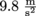, regardless of the object's mass. Both apples will land on the ground at exactly the same time as long as they are both dropped from an equal height.

Give it a try with any two objects not subject to air resistance.

4) Describe the life span of a star with a large mass relative to a star with a smaller mass.

A star needs to reach extremely high temperatures for nuclear fusion to take place. This high temperature can be reached as a star collapses on itself and shrinks. As its volume is reduced, temperature increases. The more massive a star, the more easily this process can occur.

However since nuclear fusion is much easier to achieve with more mass, the massive star burns its fuel more quickly,and will actually live a shorter life. A lighter star will not go through as many phases but last longer, such that the oldest stars in the universe today are actually stars with low masses.

5) Investigate the concept of hydrostatic equilibrium by analyzing a ball of density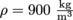and volume V = 1 m3 under water.

We know an object will displace its own volume when dropped in water, and we don't have to get naked to prove it either. We also know a buoyant force equal to the weight of water displacement will act upwards on the ball. The ball has a volume of 1 m3, which weighs 1000 kg. The buoyant force is then given by Fb = (1000)(9.8) = 9800 N.

Now since, the ball has a density ofand a volume of 1 m3, its mass is given by m = (ρ)(V) = 900 kg. So the force of gravity Fg = mg = 8820 N.

Obviously 8820 N < 9800 N, so we know the ball doesn't sink because the ball is not in equilibrium, with the buoyant force larger.

Determining its exact location from the surface will be investigated in Problem 10.

6) What changes will result in the configuration of a hydraulic lift with areas A1 and A2 if you double A1 but keep A2 and F2 (the "car side" of the lift) identical?

Here we can use Pascal's Law, which states that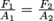.

If we increase A1, then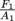decreases as a result. However, F2/A2 must stay constant. F1 is always equal to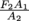. If A1 is replaced by 2A1, then the new force F1 that you need to apply to your piston area doubles.

7) Explain the physics of stalling an airplane.

We've discussed how air particles have a higher velocity while flowing over the curve of an airplane wing. We also know from Bernoulli's equation that a fluid undergoing acceleration means the pressure has to decrease. This is what causes lift.

If the air particles' flow is disrupted, however, then we start to have problems. This can happen in several situations, like ice building up on a wing, or a treacherous gust of wind taking the pilot by surprise. When airflow doesn't flow smoothly, a wing "stalls", or loses lift. The force of gravity is largest then, which 'smells like trouble since we're talking about a plane here.

8) Explain why helium balloons float.

The density of air at sea level is approximately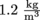. This density decreases as altitude increases, since the molecules high in the atmosphere experience less gravity. We've discussed this previously with hikers puffing and huffing as they climb Mount Everest and experience a decrease of oxygen levels. The density of helium is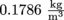.

Since helium has a lower density than air, helium balloons float. Guess what else? Eventually the helium balloon may rise to the same density in the atmosphere, but no higher.

9) Why is it impossible for a fluid to accelerate towards a smaller area and simultaneously increase its pressure?

If a fluid is accelerating, then it is under the influence of a force since F = ma. We've seen that this force actually comes from the fluid itself. Pressure is given by force divided by area, or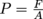. Since area A decreases, this means that pressure P increases.

From a non-math angle, we can reason that an object is lazy (remember the couch potato law), and will, in a way, always choose the easiest path if it absolutely needs to move. This concept applies to all fields of physics, whether it's light traveling or electricity running through a circuit. Keeping this in mind, we can see how a fluid would never choose to travel towards an area of high pressure, because a lot more energy would be required for the fluid.